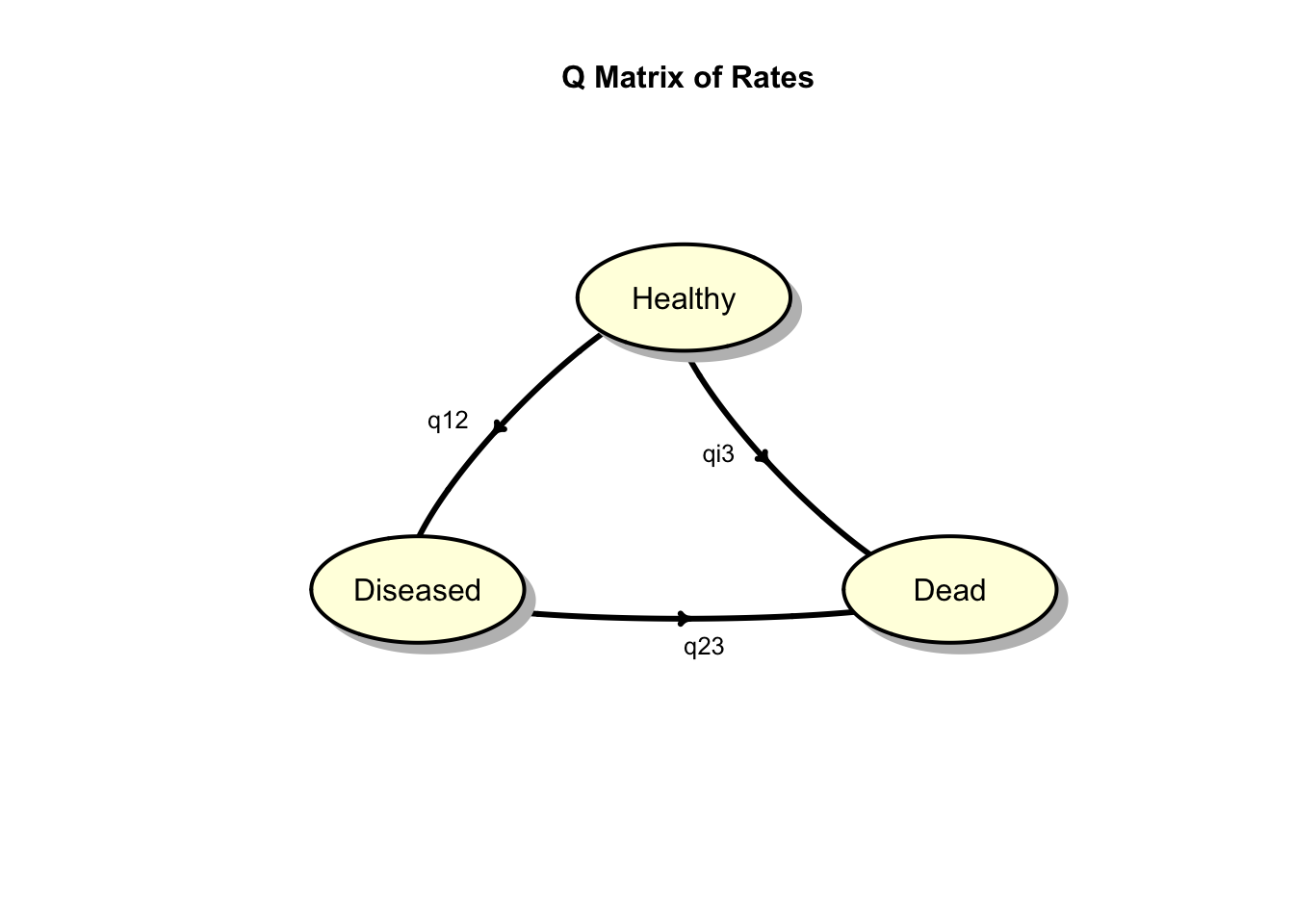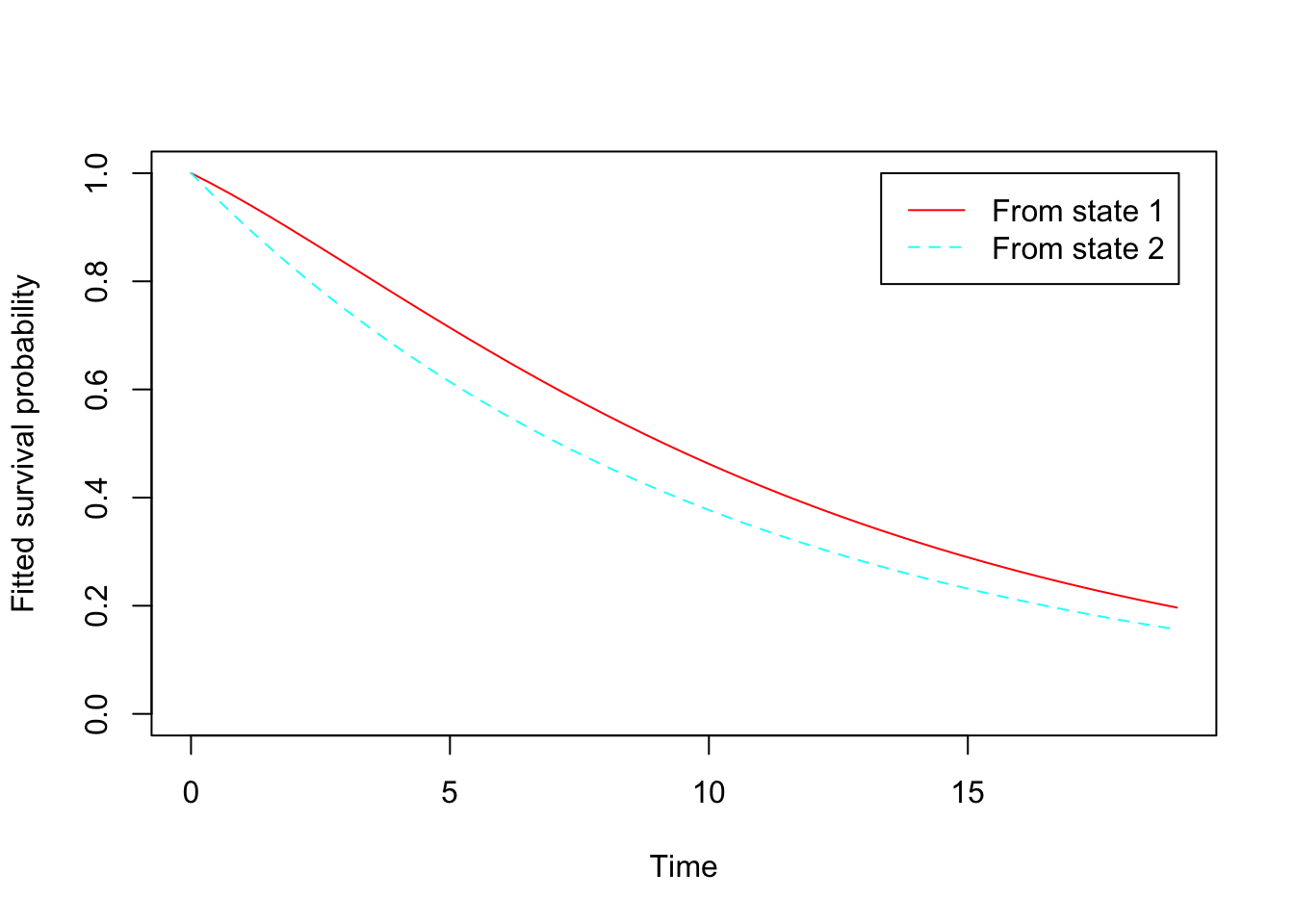# Fake Survival Data for the Disease Progression Model

by Joseph Rickert

In a previous post, I showed some examples of simulating fake data from a few packages that are useful for common simulation tasks and indicated that I would be following up with a look at simulating survival data. A tremendous amount of work in survival analysis has been done in R1 and it will take some time to explore what’s out there. In this first post, I am just going to jump into the ocean of ideas and see if I can fish out and interesting example.

Markov models are commonly used in Health Care Economics to model the progression of a disease, and the efficacy and potential benefits of various treatments. One popular approach is to consider cohorts of patients who move through the three states of being healthy (no disease progression), diseased (some level of disease progression) and dead.

The following figure illustrates the process. (I will explain the labeling on the arrows below).These kinds of models are commonly called multi-state models in the survival literature. In the simplest case, disease progression might be modeled as a discrete time Markov chain where patients move from state-to-state according to a matrix of transition probabilities which govern how the process develops at discrete time intervals. However, for many studies, limiting transitions to discrete, uniform intervals is a little too simplistic. For example, in most cases, the exact time when a patient “progresses” from healthy to deceased is not observed. To account for this, modelers frequently consider Continuous Time Markov Chain which allow modeling the distribution of time spent in each state as well as the state-to-state transitions.

One way to define a continuous time Markov chain is as a continuous time process that takes values in a discrete state space and obeys the Markov property where the transition to a future state depends only on the present and not on the past.

A continuous-time stochastic process $$X_{t}, t \geq 0$$ with discrete state space S is a continuous-time Markov chain if: $P(X_{t+s}=j \:|\: X_{s}=i), X_u = x_u, 0 \leq u < s) = P(X_{t+s}=j \: | \: X_{s}=i)$ $\forall s,t \geq 0 \:, i, j, x_{u} \in S, \: 0 \leq u < s$ If the process does not depend on the the particular value of s (the time when the process is in state i) then it is said to be time homogeneous. For a very readable account of how the definition above along with the assumption of time homogeneity ensure both the Markov property and that the time the process spends in the various states will be exponentially distributed, see Chapter 7 of Dobrow (2016).

One more bit of theory before we get to the example: unlike discrete time Markov chains, the development of a continuous time process is not driven by a transition matrix. Instead, state transition probabilities are generated by a matrix, Q, that gives the instantaneous rates of going from one state to another. Transition probabilities for any time, t, are then calculated from Q using matrix exponentiation.

$P(t)=e^Q$ The following is the Q matrix for our three state disease progression model. Notice, that this is not a stochastic matrix: the rows sum to 0 not to 1. The basic idea is that the rate of flow into a state i is equal to the flow out of i. The final row is all zeroes in our Q matrix because death is an absorbing state and there are not transitions back to healthy from diseased.

$Q = \begin{pmatrix} \ -(q_{12} + q_{13}) & q_{12} & q_{13}) \\ \ 0 & -q_{23} & q_{23} \\ \ 0 & 0 & 0 \end{pmatrix}$

Armed with a little bit of theory, let’s see how continuous time Markov chains can be used both to simulate survival data and also to fit a model to the fake data.

### Generating Simulated Survival Data

The following is essentially the example on page 12 of the pdf for the genSurv package2 listed in the CRAN Survival Task View. This shows how to use the genTHMM() function to simulate data from a time homogeneous, continuous time Markov Chain. In the code below, the model.cens parameter indicates that censoring is accomplished via a uniform distribution over the interval [0, cens.par]. A covariate is generated by a uniform distribution over the interval [0, covar] and enters the model through the equation:

$q_{i,j} = \lambda_{i,j} exp(\beta_{i,j} \cdot v)$ where $$\lambda_{i,j}$$ is the base rate, parameter rate for the genTHMM() function and $$\beta_{i,j}$$ are the regression coefficients, beta in the function. In the code below, we use the covariate output to create a sex covariate.

set.seed(1234)
thmmdata <- genTHMM( n=100, model.cens="uniform", # censorship model
cens.par = 20,
beta = c(0.01,0.08,0.05),
covar = 1,
rate = c(0.1,0.05,0.08) )

df <- thmmdata %>% mutate(sex = if_else(covariate <= .5,0,1 ))
df <- df %>% mutate_if(is.numeric, round, 3)
head(df,11)
##    PTNUM   time state covariate sex
## 1      1  0.000     1     0.114   0
## 2      1  2.183     2     0.114   0
## 3      1  2.265     3     0.114   0
## 4      2  0.000     1     0.233   0
## 5      2  0.284     2     0.233   0
## 6      2  1.396     3     0.233   0
## 7      3  0.000     1     0.283   0
## 8      3  8.600     2     0.283   0
## 9      3 18.469     2     0.283   0
## 10     4  0.000     1     0.267   0
## 11     4  3.734     1     0.267   0

For more on the theory underlying the genSurv package have a look at the paper Meira-Mechado et al. (2009).

### Fitting the Survival Model

The code in this section fits a continuous time, Markov chain survival model to the data generated above using the msm package3 and indicates how one might go about examining the output.

First, let’s look at the transitions between states that occurred for the simulated patients.

st <- statetable.msm(state, PTNUM,data = thmmdata)
st
##     to
## from  1  2  3
##    1 28 50 22
##    2  0 25 25

We see, for example, 50 progressed to the diseased state and 22 patient went directly from being healthy to dead. 25 patients who progressed to disease, subsequently died.

Next, we set up the Q matrix of instantaneous transition rates described above,

Q <- matrix(c(0, 1, 1, 0, 0 , 1, 0, 0 , 0), nrow = 3, byrow = TRUE)
rownames(Q) <- c("S1", "S2", "S3")
colnames(Q)  <- c("S1", "S2", "S3")
Q
##    S1 S2 S3
## S1  0  1  1
## S2  0  0  1
## S3  0  0  0

fit the model, and plot the survival curves for states S1 and S2 using the “old school” pre-built plot method.

fit <- msm( state ~ time, subject=PTNUM, data = df,
qmatrix = Q, gen.inits = TRUE, covariates = ~ sex)
plot(fit)The default print method for the mode fit shows the transition intensities with the hazard ratio of the covariate.

fit
##
## Call:
## msm(formula = state ~ time, subject = PTNUM, data = df, qmatrix = Q,     gen.inits = TRUE, covariates = ~sex)
##
## Maximum likelihood estimates
## Baselines are with covariates set to their means
##
## Transition intensities with hazard ratios for each covariate
##         Baseline                    sex
## S1 - S1 -0.28724 (-0.37477,-0.2202)
## S1 - S2  0.24020 ( 0.17891, 0.3225) 0.8992 (0.49769,1.625)
## S1 - S3  0.04703 ( 0.02057, 0.1076) 0.2106 (0.04132,1.073)
## S2 - S2 -0.09756 (-0.14312,-0.0665)
## S2 - S3  0.09756 ( 0.06650, 0.1431) 0.6558 (0.30473,1.411)
##
## -2 * log-likelihood:  413.3
## [Note, to obtain old print format, use "printold.msm"]

We can get the transition rates for sex = 0,

qmatrix.msm(fit, covariates = list(sex = 0))
##    S1                          S2
## S1 -0.3583 (-0.51092,-0.25130)  0.2537 ( 0.16167, 0.39804)
## S2 0                           -0.1211 (-0.20856,-0.07037)
## S3 0                           0
##    S3
## S1  0.1046 ( 0.04986, 0.21962)
## S2  0.1211 ( 0.07037, 0.20856)
## S3 0

and for sex = 1.

qmatrix.msm(fit, covariates = list(sex = 1))
##    S1                            S2
## S1 -0.25013 (-0.357720,-0.17490)  0.22810 ( 0.155472, 0.33464)
## S2 0                             -0.07945 (-0.136411,-0.04627)
## S3 0                             0
##    S3
## S1  0.02204 ( 0.005169, 0.09396)
## S2  0.07945 ( 0.046269, 0.13641)
## S3 0

and msm also allows us to calculate the transition function $$P(t)$$ for arbitrary times.

pmatrix.msm(fit, t= 13.3)
##         S1     S2     S3
## S1 0.02192 0.3182 0.6599
## S2 0.00000 0.2732 0.7268
## S3 0.00000 0.0000 1.0000

Finally, we look at the mean sojourn times for patients in the healthy and diseased states. Normally, for a process that can transition in and out of states this means the average time spent in the state each time it is visited. For our model, patients, only go forward through the chain, there is no getting better, so the sojourn for S2 is essentially the average amount of time patients spent in the diseased state.

sojourn.msm(fit)
##    estimates     SE     L      U
## S1     3.481 0.4725 2.668  4.542
## S2    10.251 2.0045 6.987 15.038

### A Few Remarks

1The work done in R on survival analysis, and partially embodied in the two hundred thirty-three packages listed in the CRAN Survival Analysis Task View, constitutes a fundamental contribution to statistics. There is enough material here for a lifetime of study. Even confining oneself to a tour of the eleven packages listed in the simulation section would be a significant undertaking.

2 genSurv is a pretty bare bones package having just seven functions and little explanatory text. If it was not listed on the CRAN Task View, it would have been easy pass by. Nevertheless, I have only shown a small portion of what it can do.

3msm is an example of why R is a treasury of statistical knowledge. Not only does the package offer an impressive array of capabilities for analyzing multi-state Markov models in continuous time, the basic documentation, the package’s pdf, includes references to quite a few of the fundamental papers.

Share Comments · · · · · · · ·